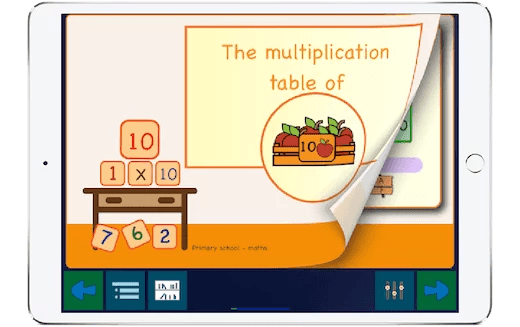Select Page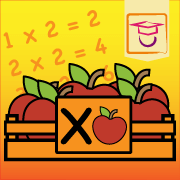# Mutliplication Tables & Apples app

## Learn times tables with boxes full of apples!

Learning the tables has never been so instructive with this app. The app contains 12 interactive workbooks with exercises for each multiplication table. First, you learn how to multiply with crates filled with apples. Next, you discover that counting with boxes is actually turning into multiplications sums with apples.

For example: how many apples are in two boxes with two apples in each box? You will learn to write this as a sum 2×2, and this will be equal to 4.

In each book, you can practice on four levels. First, you discover the story behind multiplying by a specific number. We visualize this with crates containing apples.

During the second level, you learn the tables to memorize in ascending order. At this level, we have an exercise for you, in which you train the table from one to ten. This level also contains a fun test in which you can only check the answer afterwards. We also teach you secret tricks by using funny instruction cards. You can use these tricks while practising, in case you don’t know the answer anymore.

On the third level, you will learn to solve the multiplications in random order. We have also added a helpful listening exercise. The digital teacher says the sum, and you give the correct answer. By listening, you activate another part of your learning ability, which helps you to remember the multiplication sums better.

On the fourth and last level, you learn to use the multiplications in the real world. We have created fun puzzles in which the sums are hidden. This way, you learn to use the tables in everyday life.

Finally, you close each booklet with a test, in which you make the sums within a specific time.

In this way, you learn to remember the multiplication tables 1 to 12 in a fun, quiet, interactive and educational way. In total, this app contains 108 exercises and tests to train the multiplication tables 1 to 12.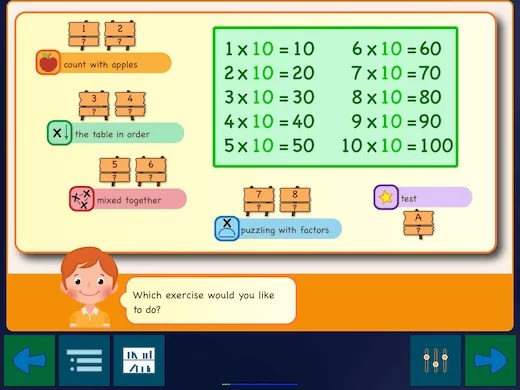### An educative app with twelve booklets

This multiplication times table app contains twelve digital practice books. Each booklet covers one table and includes eight exercises and a test.### First learn the table with apples in crates

First, you learn to understand the multiplication table by counting apples in a bucket. This way, you learn the concept of multiplying.### Learning the table in order

First, you learn the multiplication table in order. All sums are ordered from low to high. In this way, you will learn to remember the ten multiplication sums of the table.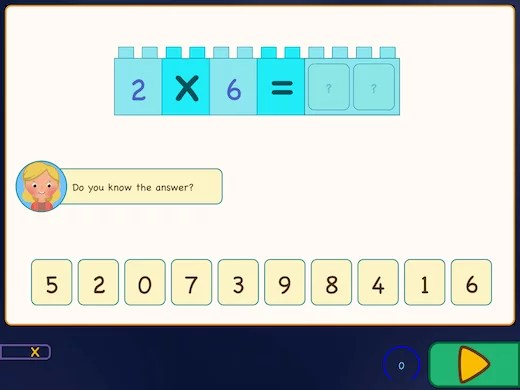### Learn the multiplications separately

When you did learn the multiplications in order, you will start to learn the sums in any order. You always make a choice by dragging the correct nummers to the correct answer boxes.### Learn to listen to the sum

The digital teacher says the sum, and you give the correct answer. By listening, you activate another part of your learning ability, which helps you to remember the multiplication sums better.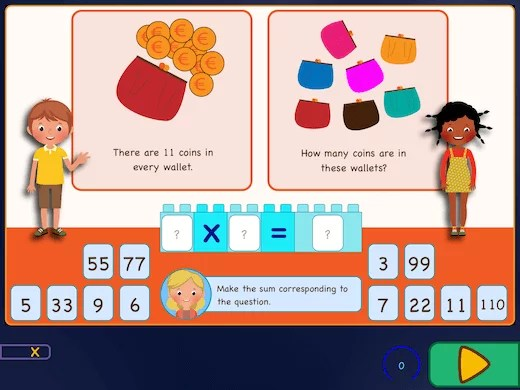### Finish the sum

In the last two exercises you play with the sums. In these exercises you get either puzzles to solve or problems in which the multiplication table plays a role. You have to make the sums by yourself.